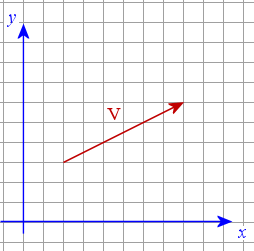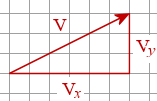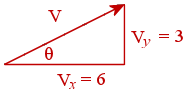Search IntMath
Close

# 3. Vectors in 2 Dimensions

So far we have considered 1-dimensional vectors only.

Now we extend the concept to vectors in 2-dimensions. We can use the familiar x-y coordinate plane to draw our 2-dimensional vectors.The vector V shown above is a 2-dimensional vector drawn on the x-y plane.

The vector V is acting in 2 different directions simultaneously (to the right and in the up direction). We can see that it has an x-component (6 units to the right) and a y-component (3 units up).

## Components of Vectors

Reading from the diagram above, the x-component of the vector V is 6 units.

The y-component of the vector V is 3 units.We can write these vector components using subscripts as follows:

Vx = 6 units

Vy = 3 units

## Magnitude of a 2-dimensional Vector

The magnitude of a vector is simply the length of the vector. We can use Pythagoras' Theorem to find the length of the vector V above.

Recall (from Section 1, Vector Concepts) that we write the magnitude of V using the vertical lines notation | V |.

We have:

 Magnitude of V = | bbV |  = sqrt(6^2+ 3^2) = sqrt(45) = 6.71\ "units"

## Direction of a 2-dimensional Vector

To describe the direction of the vector, we normally use degrees (or radians) from the horizontal, in an anti-clockwise direction.

We use simple trigonometry to find the angle. In the above example, we know the opposite (3 units) and the adjacent (6 units) values for the angle (θ) we need.So we have:

tan theta=3/6=0.5

This gives:

 θ = arctan 0.5 = 26.6° (= 0.464 radians)

So our vector has magnitude 6.71 units and direction 26.6° up from the right horizontal axis.

## Problem SolverThis tool combines the power of mathematical computation engine that excels at solving mathematical formulas with the power of GPT large language models to parse and generate natural language. This creates math problem solver thats more accurate than ChatGPT, more flexible than a calculator, and faster answers than a human tutor. Learn More.